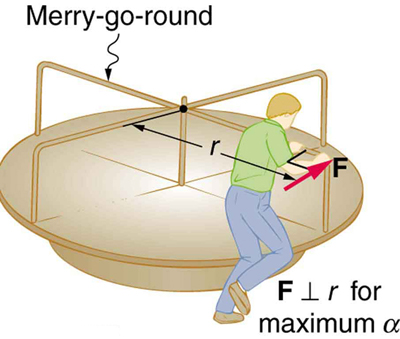# 10.3 Dynamics of rotational motion: rotational inertia  (Page 3/8)

 Page 3 / 8

## Problem-solving strategy for rotational dynamics

1. Examine the situation to determine that torque and mass are involved in the rotation . Draw a careful sketch of the situation.
2. Determine the system of interest .
3. Draw a free body diagram . That is, draw and label all external forces acting on the system of interest.
4. Apply , the rotational equivalent of Newton’s second law, to solve the problem . Care must be taken to use the correct moment of inertia and to consider the torque about the point of rotation.
5. As always, check the solution to see if it is reasonable .

## Making connections

In statics, the net torque is zero, and there is no angular acceleration. In rotational motion, net torque is the cause of angular acceleration, exactly as in Newton’s second law of motion for rotation.

## Calculating the effect of mass distribution on a merry-go-round

Consider the father pushing a playground merry-go-round in [link] . He exerts a force of 250 N at the edge of the 50.0-kg merry-go-round, which has a 1.50 m radius. Calculate the angular acceleration produced (a) when no one is on the merry-go-round and (b) when an 18.0-kg child sits 1.25 m away from the center. Consider the merry-go-round itself to be a uniform disk with negligible retarding friction.A father pushes a playground merry-go-round at its edge and perpendicular to its radius to achieve maximum torque.

Strategy

Angular acceleration is given directly by the expression $\alpha =\frac{\text{net τ}}{I}$ :

$\alpha =\frac{\tau }{I}.$

To solve for $\alpha$ , we must first calculate the torque $\tau$ (which is the same in both cases) and moment of inertia $I$ (which is greater in the second case). To find the torque, we note that the applied force is perpendicular to the radius and friction is negligible, so that

$\mathrm{\tau }=\mathrm{rF}\phantom{\rule{0.25em}{0ex}}\text{sin θ}=\left(\text{1.50 m}\right)\left(\text{250 N}\right)=\text{375 N}\cdot \text{m.}$

Solution for (a)

$\frac{1}{2}{\text{MR}}^{2}\text{,}$

where $M=\text{50.0 kg}$ and $R=\text{1.50 m}$ , so that

$I=\left(0\text{.}500\right)\left(\text{50.0 kg}\right)\left(\text{1.50 m}{\right)}^{2}=\text{56.25 kg}\cdot {\text{m}}^{2}\text{.}$

Now, after we substitute the known values, we find the angular acceleration to be

$\alpha =\frac{\tau }{I}=\frac{\text{375 N}\phantom{\rule{0.25em}{0ex}}\text{⋅}\phantom{\rule{0.25em}{0ex}}\text{m}}{\text{56.25 kg}\cdot {\text{m}}^{2}}=\text{6.67}\frac{\text{rad}}{{\text{s}}^{2}}\text{.}$

Solution for (b)

We expect the angular acceleration for the system to be less in this part, because the moment of inertia is greater when the child is on the merry-go-round. To find the total moment of inertia $I$ , we first find the child’s moment of inertia ${I}_{\text{c}}$ by considering the child to be equivalent to a point mass at a distance of 1.25 m from the axis. Then,

${I}_{\text{c}}={\mathrm{MR}}^{2}=\left(\text{18.0 kg}\right)\left(\text{1.25 m}{\right)}^{2}=\text{28.13 kg}\cdot {\text{m}}^{2}\text{.}$

The total moment of inertia is the sum of moments of inertia of the merry-go-round and the child (about the same axis). To justify this sum to yourself, examine the definition of $I$ :

$I=\text{28.13 kg}\cdot {\text{m}}^{2}+\text{56.25 kg}\cdot {\text{m}}^{2}=\text{84.38 kg}\cdot {\text{m}}^{2}.$

Substituting known values into the equation for $\alpha$ gives

$\alpha =\frac{\text{τ}}{I}=\frac{\text{375 N}\cdot \text{m}}{\text{84.38 kg}\cdot {\text{m}}^{2}}=\text{4.44}\frac{\text{rad}}{{\text{s}}^{2}}\text{.}$

Discussion

The angular acceleration is less when the child is on the merry-go-round than when the merry-go-round is empty, as expected. The angular accelerations found are quite large, partly due to the fact that friction was considered to be negligible. If, for example, the father kept pushing perpendicularly for 2.00 s, he would give the merry-go-round an angular velocity of 13.3 rad/s when it is empty but only 8.89 rad/s when the child is on it. In terms of revolutions per second, these angular velocities are 2.12 rev/s and 1.41 rev/s, respectively. The father would end up running at about 50 km/h in the first case. Summer Olympics, here he comes! Confirmation of these numbers is left as an exercise for the reader.

full meaning of GPS system
how to prove that Newton's law of universal gravitation F = GmM ______ R²
sir dose it apply to the human system
prove that the centrimental force Fc= M1V² _________ r
prove that centripetal force Fc = MV² ______ r
Kaka
how lesers can transmit information
griffts bridge derivative
below me
please explain; when a glass rod is rubbed with silk, it becomes positive and the silk becomes negative- yet both attracts dust. does dust have third types of charge that is attracted to both positive and negative
what is a conductor
Timothy
hello
Timothy
below me
why below you
Timothy
no....I said below me ...... nothing below .....ok?
dust particles contains both positive and negative charge particles
Mbutene
corona charge can verify
Stephen
when pressure increases the temperature remain what?
remains the temperature
betuel
what is frequency
define precision briefly
CT scanners do not detect details smaller than about 0.5 mm. Is this limitation due to the wavelength of x rays? Explain.
hope this helps
what's critical angle
The Critical Angle Derivation So the critical angle is defined as the angle of incidence that provides an angle of refraction of 90-degrees. Make particular note that the critical angle is an angle of incidence value. For the water-air boundary, the critical angle is 48.6-degrees.
okay whatever
Chidalu
pls who can give the definition of relative density?
Temiloluwa
the ratio of the density of a substance to the density of a standard, usually water for a liquid or solid, and air for a gas.
Chidalu
What is momentum
mass ×velocity
Chidalu
it is the product of mass ×velocity of an object
Chidalu
how do I highlight a sentence]p? I select the sentence but get options like copy or web search but no highlight. tks. src
then you can edit your work anyway you want
Wat is the relationship between Instataneous velocity
Instantaneous velocity is defined as the rate of change of position for a time interval which is almost equal to zero
AstronomyByByBy Ellie BanfieldBy Stephen VoronBy OpenStaxBy OpenStaxBy RhodesBy Robert MorrisBy Sarah WarrenBy OpenStaxBy Brenna FikeBy Madison Christian